# 2. DoreFa-Net¶

## 2.1 比特卷积核¶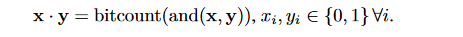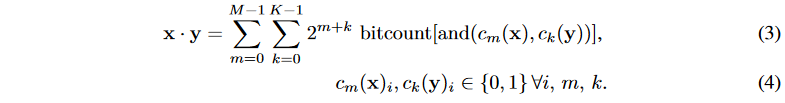在上面的等式中，计算复杂度为$M*K$，和位宽成正比。

## 2.2 直通估计器¶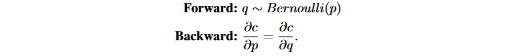## 2.3 权重的低比特量化¶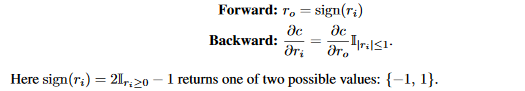$k>1$时，论文使用$k$位表达的权重，然后将STE $f_{w}^k$应用在权重上：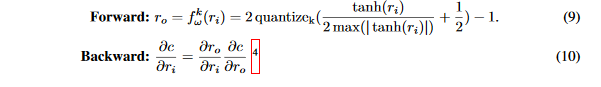$quantize_k=\frac{1}{2^k-1}round((2^k-1)r_i)$

## 2.4 梯度的低比特量化¶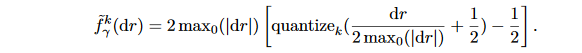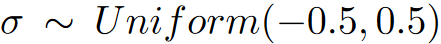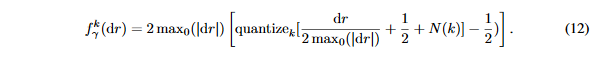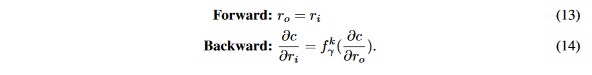## 2.5 DoReFa-Net训练算法¶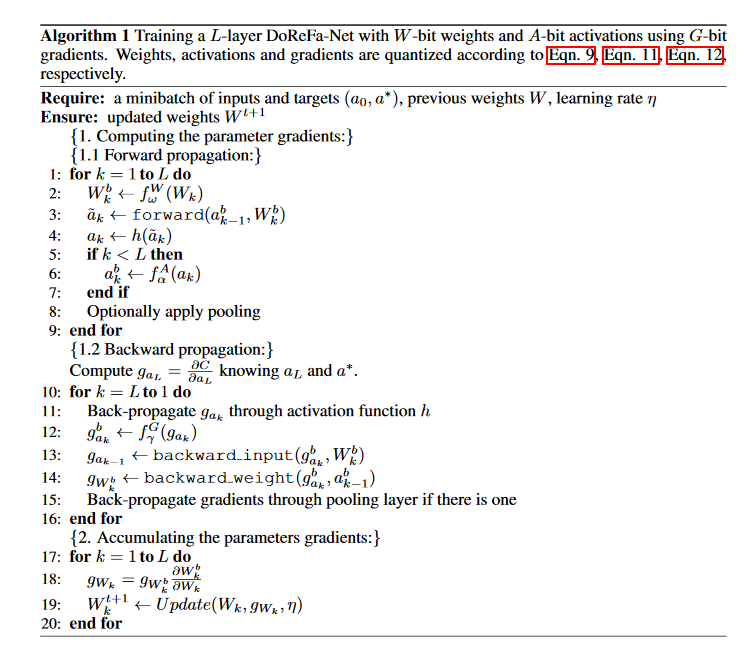# 3. 代码实战¶

https://github.com/666DZY666/model-compression


# 注意这个代码中对卷积层和全连接层实现了DoreFa-Net的量化方法
import torch
import torch.nn as nn
import torch.nn.functional as F
from .util_wqaq import Conv2d_Q

class DorefaConv2d(nn.Module):
def __init__(self, input_channels, output_channels,
kernel_size=-1, stride=-1, padding=-1, groups=1, last_relu=0, abits=8, wbits=8, first_layer=0):
super(DorefaConv2d, self).__init__()
self.last_relu = last_relu
self.first_layer = first_layer

self.q_conv = Conv2d_Q(input_channels, output_channels,
# BN和激活都保留了常规方式
self.bn = nn.BatchNorm2d(output_channels)
self.relu = nn.ReLU(inplace=True)

def forward(self, x):
# 第一个卷积层后面不接relu激活函数
if not self.first_layer:
x = self.relu(x)
x = self.q_conv(x)
x = self.bn(x)
# 最后一层卷积层后要使用relu激活函数
if self.last_relu:
x = self.relu(x)
return x

class Net(nn.Module):
def __init__(self, cfg = None, abits=8, wbits=8):
super(Net, self).__init__()
if cfg is None:
# 网络层通道数
cfg = [192, 160, 96, 192, 192, 192, 192, 192]

# model - A/W全量化(除输入、输出外)
self.dorefa = nn.Sequential(
DorefaConv2d(3, cfg, kernel_size=5, stride=1, padding=2, abits=abits, wbits=wbits, first_layer=1),
DorefaConv2d(cfg, cfg, kernel_size=1, stride=1, padding=0, abits=abits, wbits=wbits),
DorefaConv2d(cfg, cfg, kernel_size=1, stride=1, padding=0, abits=abits, wbits=wbits),

DorefaConv2d(cfg, cfg, kernel_size=5, stride=1, padding=2, abits=abits, wbits=wbits),
DorefaConv2d(cfg, cfg, kernel_size=1, stride=1, padding=0, abits=abits, wbits=wbits),
DorefaConv2d(cfg, cfg, kernel_size=1, stride=1, padding=0, abits=abits, wbits=wbits),

DorefaConv2d(cfg, cfg, kernel_size=3, stride=1, padding=1, abits=abits, wbits=wbits),
DorefaConv2d(cfg, cfg, kernel_size=1, stride=1, padding=0, abits=abits, wbits=wbits),
DorefaConv2d(cfg, 10, kernel_size=1, stride=1, padding=0, last_relu=1, abits=abits, wbits=wbits),
)

def forward(self, x):
x = self.dorefa(x)
x = x.view(x.size(0), -1)
return x


# ********************* 量化卷积（同时量化A/W，并做卷积） ***********************
class Conv2d_Q(nn.Conv2d):
def __init__(
self,
in_channels,
out_channels,
kernel_size,
stride=1,
dilation=1,
groups=1,
bias=True,
a_bits=8,
w_bits=8,
first_layer=0
):
super().__init__(
in_channels=in_channels,
out_channels=out_channels,
kernel_size=kernel_size,
stride=stride,
dilation=dilation,
groups=groups,
bias=bias
)
# 实例化调用A和W量化器
self.activation_quantizer = activation_quantize(a_bits=a_bits)
self.weight_quantizer = weight_quantize(w_bits=w_bits)
self.first_layer = first_layer

def forward(self, input):
# 量化A和W
if not self.first_layer:
input = self.activation_quantizer(input)
q_input = input
q_weight = self.weight_quantizer(self.weight)
# 量化卷积
output = F.conv2d(
input=q_input,
weight=q_weight,
bias=self.bias,
stride=self.stride,
dilation=self.dilation,
groups=self.groups
)
return output


# ********************* W(模型参数)量化 ***********************
class weight_quantize(nn.Module):
def __init__(self, w_bits):
super().__init__()
self.w_bits = w_bits

def round(self, input):
output = Round.apply(input)
return output

def forward(self, input):
if self.w_bits == 32:
output = input
elif self.w_bits == 1:
print('！Binary quantization is not supported ！')
assert self.w_bits != 1
else:
# 按照公式9和10计算
output = torch.tanh(input)
output = output / 2 / torch.max(torch.abs(output)) + 0.5  #归一化-[0,1]
scale = float(2 ** self.w_bits - 1)
output = output * scale
output = self.round(output)
output = output / scale
output = 2 * output - 1
return output


class Round(Function):

@staticmethod
def forward(self, input):
output = torch.round(input)
return output

@staticmethod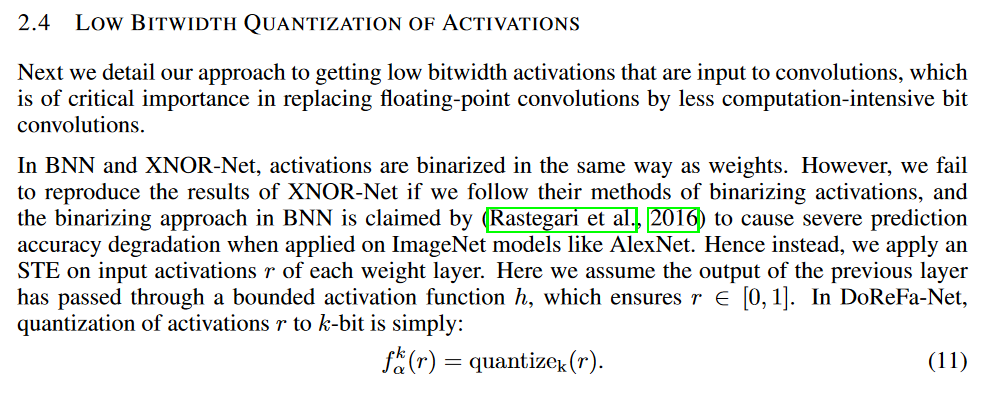# ********************* A(特征)量化 ***********************
class activation_quantize(nn.Module):
def __init__(self, a_bits):
super().__init__()
self.a_bits = a_bits

def round(self, input):
output = Round.apply(input)
return output

def forward(self, input):
if self.a_bits == 32:
output = input
elif self.a_bits == 1:
print('！Binary quantization is not supported ！')
assert self.a_bits != 1
else:
output = torch.clamp(input * 0.1, 0, 1)  # 特征A截断前先进行缩放（* 0.1），以减小截断误差
scale = float(2 ** self.a_bits - 1)
output = output * scale
output = self.round(output)
output = output / scale
return output


# ********************* 量化全连接（同时量化A/W，并做全连接） ***********************
class Linear_Q(nn.Linear):
def __init__(self, in_features, out_features, bias=True, a_bits=2, w_bits=2):
super().__init__(in_features=in_features, out_features=out_features, bias=bias)
self.activation_quantizer = activation_quantize(a_bits=a_bits)
self.weight_quantizer = weight_quantize(w_bits=w_bits)

def forward(self, input):
# 量化A和W
q_input = self.activation_quantizer(input)
q_weight = self.weight_quantizer(self.weight)
# 量化全连接
output = F.linear(input=q_input, weight=q_weight, bias=self.bias)
return output


# 4. 将DoreFa-Net应用到YOLOV3上¶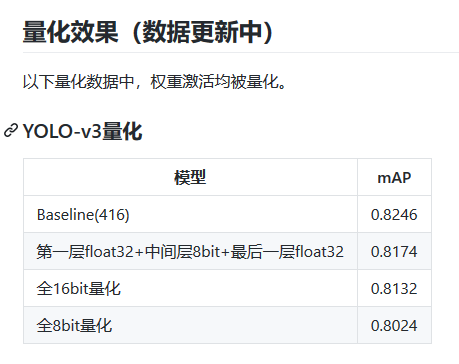# 5. 总结¶